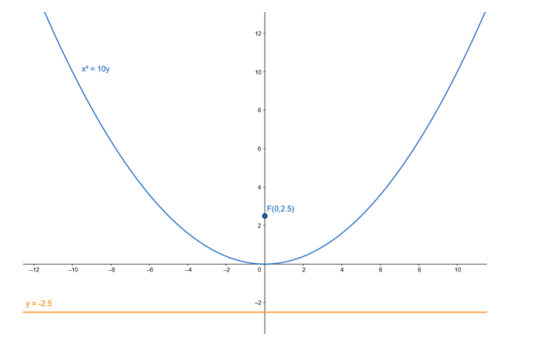# Find the coordinates of the focus and the vertex, the equations of the`
Question:

Find the coordinates of the focus and the vertex, the equations of the directrix and the axis, and length of the latus rectum of the parabola :

$x^{2}=10 y$

Solution:

Given equation : $x^{2}=10 y$

Comparing given equation with parabola having equation

$x^{2}=4 a y$

$4 a=10$

$\cdot a=2.5$

Focus : $F(0, a)=F(0,2.5)$

Vertex : $A(0,0)=A(0,0)$

Equation of the directrix : $y+a=0$

$-y+2.5=0$

$\cdot y=-2.5$

Lenth of latusrectum : 4a = 10